# Model selection in RToday in R Club we’ll be talking about model selection. My plan is to go through and explain Max Kuhn’s caret tutorial for model training and tuning. Max Kuhn is a machine learning pro at Pfizer who wrote the caret package and the free-to-download (through the UO library) super popular book, Applied Predictive Modeling.

Also, I gathered some cool data from draftexpress.com that we can use to practice on if we have time. Here is the data problem:

Every summer, 60 of the best basketball players in the world are drafted into the National Basketball Association (NBA). The majority of the draftees are selected from among thousands of eligible NCAA-level college basketball players.
Who will be drafted?

If you are not a sports fan, you can still appreciate the data if you like numbers. Get the draft data.

# Network Mapping tool from earlier in the term

I’ve finally written a full report on the R-based network mapping tool that I presented earlier this term. If you’re interested, you can find it here.

# LOOCV

LOOCV (leave one out cross validation)

Download this excellent book: http://www-bcf.usc.edu/~gareth/ISL/ and search “LOOCV” or just scroll to section 5.1.2. on p. 178.

Here’s the dataset we’re using: http://www-bcf.usc.edu/~gareth/ISL/Auto.csv

```# LOOCV (leave one out cross validation) install.packages("boot") require(boot) ?cv.glm```

``` # cv.glm(data, glmfit, cost, K) # this runs k-fold cross validation. When k = the number of observations in your dataset, then that's LOOCV # to run LOOCV, set k=n or just don't specify (its default is k=n) # cost specifies how to evaluate how good the model is; the default is the average squared error function. # it requires a glm object as input, so you need to run your model first and then validate it. ```

```Auto <- read.csv("http://www-bcf.usc.edu/~gareth/ISL/Auto.csv") str(Auto) # uh-oh. it interpreted horsepower as a factor, but it shoudl be numeric. Auto\$horsepower <- as.numeric(Auto\$horsepower) # run a simple regression, predicting mpg from horsepower model1 <- glm(mpg ~ horsepower, data=Auto) summary(model1) # okay, now let's cross-validate that model. # note: this takes a long time! it's running nearly 400 models. if you want it to be faster, you can set k to something smaller than n loocv1 <- cv.glm(data=Auto, glmfit=model1) # the only thing you really need in this loocv is an object called delta. it's got two items in it. The first component is the raw cross-validation estimate of prediction error. The second component is the adjusted cross-validation estimate. The adjustment is designed to compensate for the bias introduced by not using leave-one-out cross-validation. Since we ran LOOCV, the two values should be super similar. loocv1\$delta # okay great. we have an estimate of model fit for the simple linear regession. let's play around with adding polynomial terms and see if we can improve the fit. # there's a handy way to add polynomial terms using poly() model2 <- glm(mpg ~ poly(horsepower, 2), data=Auto) model3 <- glm(mpg ~ poly(horsepower, 3), data=Auto) model4 <- glm(mpg ~ poly(horsepower, 4), data=Auto) model5 <- glm(mpg ~ poly(horsepower, 5), data=Auto) model6 <- glm(mpg ~ poly(horsepower, 6), data=Auto) # i'm going to switch from LOOCV to a 20-fold cv, to save a little processing time squared_errs <- rep(0,6) loocv <- cv.glm(data=Auto, glmfit=model1, K=20) squared_errs <- loocv\$delta loocv <- cv.glm(data=Auto, glmfit=model2, K=20) squared_errs <- loocv\$delta loocv <- cv.glm(data=Auto, glmfit=model3, K=20) squared_errs <- loocv\$delta loocv <- cv.glm(data=Auto, glmfit=model4, K=20) squared_errs <- loocv\$delta loocv <- cv.glm(data=Auto, glmfit=model5, K=20) squared_errs <- loocv\$delta loocv <- cv.glm(data=Auto, glmfit=model6, K=20) squared_errs <- loocv\$delta # I'm sure there's a way better way to do that with a for loop or something, but i couldn't get it to work. # this is a vector of the squared error for each model squared_errs # let's plot it to get a sense for how fit changes as a function of polynomial order plot(squared_errs) # looks like there's a big drop in error when we go from 2nd order to 3rd order (i.e. allowing a cubic function) # that makes sense, given the data: plot(Auto\$mpg~Auto\$horsepower) # looks cubic # pretty plotting time :) library(ggplot2) library(RColorBrewer) ggplot(data=Auto, aes(x=horsepower, y=mpg)) + geom_point() # to plot the lines from each function, use predict() to get the predicted values from each regression equation Auto\$model1 <- predict(model1) Auto\$model2 <- predict(model2) Auto\$model3 <- predict(model3) Auto\$model4 <- predict(model4) Auto\$model5 <- predict(model5) Auto\$model6 <- predict(model6) # pick a palette display.brewer.all() colors <- brewer.pal(9,"Spectral") colors <- c(colors[1:3], colors[7:9]) #take just the 6 colors from the two ends (they're brighter) # how to check out a color palette n <- 6 # the number of colors to plot pie(rep(1,n), col=colors) # plot all of the 6 models on the data. notice that the model doesn't improve much after cubic. ggplot(data=Auto, aes(x=horsepower, y=mpg)) + geom_point() + geom_line(aes(x=horsepower, y=model1, colour="linear"), size = 2, alpha = .5 ) + geom_line(aes(x=horsepower, y=model2, colour="quadratic"), size = 2, alpha = .5 ) + geom_line(aes(x=horsepower, y=model3, colour="cubic"), size = 2, alpha = .5 ) + geom_line(aes(x=horsepower, y=model4, colour="quartic"), size = 2, alpha = .5 ) + geom_line(aes(x=horsepower, y=model5, colour="fiveish"), size = 2, alpha = .5 ) + geom_line(aes(x=horsepower, y=model6, colour="sixtacular"), size = 2, alpha = .5 ) + guides(color=guide_legend(title="model")) ```

# Nice Tables from R Data Frames

The knitr package provides the kable function, which allows you to export data frames as HTML, markdown, and more. It’s really useful along with some background with LaTeX or HTML/CSS to make nicely formatted tables directly from your R output. The code below should get you started.

(Bonus: a brief look at plyr power!)

``` #install.packages('knitr') require(knitr)```

``` #lets use the apropos Number of Breaks in Yarn during Weaving data data(warpbreaks) #check it out summary(warpbreaks) #Let's get the mean and SD for each level of wool # at each level of tension. #install.packages('plyr') # This is a great package require(plyr) #Split the data by wool and tension, get mean and sd for each # and return a data frame. descriptives<-ddply(.data=warpbreaks, .variables=c('wool','tension'), .fun=function(x){ round(c(Mean=mean(x\$breaks),SD=sd(x\$breaks)),2) }) descriptives #The default is markdown, and looks pretty good to copy and # past into a text file. kable(descriptives) ```

```myHTMLTable<-kable(descriptives,format='html',output=F) getwd() #what directory will it save into? write(myHTMLTable,file='table.html') #Check out the html, and buff it up if you want! #Further reading: # ?kable # ?plyr # Also, google 'markdown' and 'pandoc' ```

This is the html output:

wool tension Mean SD
A L 44.56 18.10
A M 24.00 8.66
A H 24.56 10.27
B L 28.22 9.86
B M 28.78 9.43
B H 18.78 4.89

# Power analysis (and other stuff)!

```# R CLUB 5/15: Power Analyses in R ## Brendan Ostlund```

``` # REFRESHER: POWER is the probability of correctly rejecting a null hypothesis that is actually false. # P(rejecting NULL hypothesis|NULL hypothesis is false) # In other words, its the prob. of finding an effect that is really present. # Power = 1 - P(Type II error) # Power, effect size, sample size, and the significance level are inter-related, and if you know 3 of these # quantities you can calculate the fourth (exciting eh?). Let's get to it. #First, we'll need to install the "pwr" package. install.packages("pwr") library(pwr) # Calculating Cohen's effect size (Rules of Thumb) in case we werent able to remember. # select the stats test of interest and one effect size from: cohen.ES(test = c("p", "t", "r", "anov", "chisq", "f2"), size = c("small", "medium", "large")) # to determine effect size according to Cohen's Rules of Thumb. # For example, a large effect for a multiple regression would be: cohen.ES(test = c("f2"), size = c("large")) # whereas a small effect for anova would be: cohen.ES(test = c("chisq"), size = c("medium")) #POWER ANALYSIS FUNCTIONS! # T-TESTS! # Function: pwr.t.test(n = , d = , sig.level = , power = , type = c("two.sample", "one.sample", "paired"), alternative= c("two.sided", "less", "greater")) # Remember, power, effect size, sample size, and the significance level are related so we can play around # with the information we have to determine the missing quantity. #For example, what is the power for a two-sided ind. samples t-test given n=25 and a medium effect size? pwr.t.test(n=25, d=.5, sig.level= .05) #Another example: What is the sample size needed to achieve a pwr=.80 and a small effect size? pwr.t.test(d=.2, sig.level= .05, power=.8) # Note: if you add "\$n" to the end of the command only the necessary sample size will be displayed. pwr.t.test(d=.2, sig.level= .05, power=.8)\$n # R can also calculate pwr for two samples with unequal sample sizes: pwr.t2n.test(n1= 35, n2= 17, d=.8, sig.level= .05, alternative = "greater") # ANOVA! # what's Cohen's rules of thumb for anova effect size (i.e., "f")? cohen.ES(test = c("anov"), size = c("small")) cohen.ES(test = c("anov"), size = c("medium")) cohen.ES(test = c("anov"), size = c("large")) # One-way ANOVA pwr analysis function: pwr.anova.test(k = , n = , f = , sig.level = , power = ) # where k is the number of groups and n is the number of obs. IN EACH GROUP. pwr.anova.test(k =4, n=32, f=.3, sig.level =.05) # More complex (e.g., factorial ANOVA, ANCOVA) pwr analysis?? --> to be continued. # PROPORTIONS! # Function: pwr.2p.test(h = , n = , sig.level =, power = )              # 2 proportions with equal sample sizes pwr.2p2n.test(h = , n1 = , n2 = , sig.level = , power = )   # 2 proportions with unequal sample sizes # CORRELATION! #Function: pwr.r.test(n = , r = , sig.level = , power = ) # CHI-SQUARE # Function: pwr.chisq.test(w =, N = , df = , sig.level =, power = ) # where "w"= effect size and "n"=number of observations. # Quick example: pwr.chisq.test(w=.3, N=120, df=9, sig.level=.05) # REGRESSION! # Function: pwr.f2.test(u =, v = , f2 = , sig.level = , power = ) # where "u"= numerator degrees of freedom (number of continuous variables + number of dummy codes - 1) # and "v"=denominator (error) degrees of freedom # For example, find the power for a multiple regression test with 2 continuous predictors and 1 categorical # predictor (i.e. Marital status with k=3 so 3-1=2 dummy codes) that has a large effect size and a sample size of 30. pwr.f2.test(u =3, v =30, f2 =.35, sig.level =.05) # Generating a table of sample sizes # What is the required sample size for an independent samples t-test with pwr=.80? seq <- seq(.1,.8, .1) FindN <- rep(0,8) for(i in 1:8) FindN[i]=pwr.t.test(d=seq[i],power=.8,sig.level=.05,type="two.sample")\$n data.frame(d=seq , N=ceiling(FindN)) # can swap in other functions, just make sure arguments are relevant to test seq <- seq(.1,.8, .1) FindN <- rep(0,8) for(i in 1:8) FindN[i]=pwr.anova.test(k=4, f=seq[i], power=.8,sig.level=.05)\$n data.frame(f=seq , N=ceiling(FindN)) # NOTE: "ceiling" is an argument for rounding numbers so the values are slightly different than if we # computed the sample size based on specified values. For example... pwr.anova.test(k =4,f=.3, sig.level =.05, power=.8) # Finally, plot of sample size curves for detecting effect at various sample sizes. # range of correlations d <- seq(.1,.5,.01) nd <- length(d) # power values p <- seq(.4,.9,.1) np <- length(p) # obtain sample sizes samsize <- array(numeric(nd*np), dim=c(nd,np)) for (i in 1:np){ for (j in 1:nd){ result <- pwr.t.test(n = NULL, d = d[j], sig.level = .05, power = p[i], alternative = "two.sided") samsize[j,i] <- ceiling(result\$n) } } # set up graph xrange <- range(d) yrange <- round(range(samsize)) colors <- rainbow(length(p)) plot(xrange, yrange, type="n", xlab="Effect size (d)", ylab="Sample Size (n)" ) # add power curves for (i in 1:np){ lines(d, samsize[,i], type="l", lwd=2, col=colors[i]) } # add title and legend title("Sample Size Estimation for t-test") legend("topright", title="Power", as.character(p), fill=colors) ```

```#HELPFUL RESOURCE: # Quick-R blog: http://www.statmethods.net/stats/power.html```

# Basic Data Visualization with ggplot2

```###Basic Data Visualization with ggplot2 ###R Club - 5/1/1014 ###Joe Hoover```

``` #Let's make some data library(gridExtra) set.seed(10005) ATTEND rnorm(1500, mean = 16), rnorm(500, mean = 19)) GRADE rnorm(1500, mean = 83, sd = 4), rnorm(500, mean = 92, sd = 2)) BOOKS data #We have books read, classes attended, and grade. #We're interested in predicting student grades from #the number of books they've read, and the number #of classes they attended. ##Graphing with ggplot involves a process of specifying layers of graphic objects. #Let's start with a histogram #What does the grade distribution look like? ggplot(data, aes(GRADE)) + #The first step is to identify the data you want to graph. #In this case, we want to graph the variable GRADE, which can be found in data geom_histogram() #Now, we need to tell ggplot2 how we want the data represented. #That's not very nice. Let's eliminate the black fill: grade_hist = ggplot(data, aes(GRADE)) + geom_histogram(fill=NA, color="black") grade_hist #Let's add some lables: grade_hist + labs(x="Grades", y = "Number of Grades") #We can also display grades in a density plot: d1 = ggplot(data, aes(GRADE)) + geom_density() d1 #Color? d1 = ggplot(data, aes(GRADE, fill="red")) + geom_density() d1 #Now, what if we want to look at the density distribution of grades #broken down into categories of number of books read? d2 = ggplot(data, aes(GRADE, fill=factor(BOOKS))) + geom_density(alpha = 0.2) d2 #We probably don't need the legend... d2 = ggplot(data, aes(GRADE, fill="red")) + geom_density(alpha = 0.2) + theme(legend.position = "none") d2 #Let's look at grades by books read: b1 = ggplot(data, aes(GRADE)) + geom_density(aes(fill = factor(BOOKS))) #Here, we tell ggplot we want different colors for our factor BOOKS b1 #What about barplots? ggplot(data, aes(x = factor(BOOKS), y = GRADE)) + geom_bar(stat="identity") #What happened? Why are the grades so high? #It looks like we have summed grades, which is not particularly interesting. #Let's use stat_summary to tell ggplot we want the mean grade for each level of book read. b1 = ggplot(data, aes(x = factor(BOOKS), y=GRADE, fill = factor(BOOKS))) + stat_summary(fun.y=mean, geom="bar") + scale_fill_manual(values=c("purple", "orange", "red")) b1 #Box plots, jitter plots, and violins: b1 = ggplot(data, aes(BOOKS, GRADE)) + geom_boxplot(aes(fill=BOOKS)) b1 #jitter plot b2<-ggplot(data, aes(BOOKS, GRADE)) + geom_jitter(alpha=I(1/4), aes(color=BOOKS)) + theme(legend.position = "none") b2 #violin plot b3<-ggplot(data, aes(x = GRADE)) + stat_density(aes(ymax = ..density.., ymin = -..density.., fill = BOOKS, color = BOOKS), geom = "ribbon", position = "identity") + facet_grid(. ~ BOOKS) + coord_flip() + theme(legend.position = "none") b3 #jitter plot + boxplot b4<-ggplot(data, aes(BOOKS, GRADE)) + geom_jitter(alpha=I(1/4), aes(color=BOOKS)) + geom_boxplot(aes(fill=BOOKS)) + theme(legend.position = "none") b4 #Let's display them all together: grid.arrange(b1, b2, b3, b4, nrow=1) #Alright, how about scatterplots? #First we need to declare the data we want to graph. #Let's look at grades by attendence: s1 = ggplot(data, aes(x = ATTEND, y = GRADE)) s2 = s1 + geom_point() #add a layer of plot points s2 #Let's use BOOKSe to color the points: s2 = s1 + geom_point(aes(color=factor(BOOKS))) + scale_color_manual(values=c("purple", "orange", "red")) s2 #Let's add a regression line s2 + geom_smooth(method = "lm") + facet_grid(. ~ BOOKS) #How about regression lines for each level of books? s2 + geom_smooth(method = "lm", aes(color = factor(BOOKS))) #No error bars ggplot(data, aes(x = ATTEND, y = GRADE)) + geom_point(aes(color=factor(BOOKS))) + scale_color_manual(values=c("purple", "orange", "red")) + geom_smooth(method = "lm", se=FALSE, aes(color = factor(BOOKS))) ##Kind of hard to see, let's plot the regression lines without the data points: #No error bars ggplot(data, aes(x = ATTEND, y = GRADE)) + scale_color_manual(values=c("purple", "orange", "red")) + geom_smooth(method = "lm", se=FALSE, aes(color = factor(BOOKS))) #Combining graphs: #Let's plot several different graphs together. #We're going to use grid.arrange to do this. #First, however, we need to create an empty placeholder plot: #placeholder plot - prints nothing at all empty theme( plot.background = element_blank(), panel.grid.major = element_blank(), panel.grid.minor = element_blank(), panel.border = element_blank(), panel.background = element_blank(), axis.title.x = element_blank(), axis.title.y = element_blank(), axis.text.x = element_blank(), axis.text.y = element_blank(), axis.ticks = element_blank() ) #scatterplot of x and y variables scatter geom_point(aes(color=BOOKS)) + scale_color_manual(values = c("orange", "purple", "red")) + theme(legend.position=c(1,1),legend.justification=c(1,1)) #marginal density of x - plot on top plot_top geom_density(alpha=.5) + scale_fill_manual(values = c("orange", "purple", "red")) + theme(legend.position = "none") #marginal density of y - plot on the right plot_right geom_density(alpha=.5) + coord_flip() + scale_fill_manual(values = c("orange", "purple", "red")) + theme(legend.position = "none") plot_right #arrange the plots together, with appropriate height and width for each row and column grid.arrange(plot_top, empty, scatter, plot_right, ncol=2, nrow=2, widths=c(4, 1), heights=c(1, 4)) #scatterplot of x and y variables scatter geom_point(aes(color=BOOKS)) + scale_color_manual(values = c("orange", "purple", "red")) + theme(legend.position=c(1,1),legend.justification=c(1,1)) #marginal density of x - plot on top plot_top geom_density(alpha=.5) + scale_fill_manual(values = c("orange", "purple", "red")) + theme(legend.position = "none") + scale_y_continuous(breaks=c(0.0, .2, .4)) #Define breaks for y-axis #marginal density of y - plot on the right plot_right geom_density(alpha=.5) + coord_flip() + scale_fill_manual(values = c("orange", "purple", "red")) + theme(legend.position = "none") + scale_y_continuous(breaks=c(0.0, .1, .2)) #Define breaks for y-axis #arrange the plots together, with appropriate height and width for each row and column grid.arrange(plot_top, empty, scatter, plot_right, ncol=2, nrow=2, widths=c(4, 1), heights=c(1, 4)) ############################################## #######################RESOURCES############## ```

```##Much of the code above was adapted from this excellent blog: ##http://rforpublichealth.blogspot.com/ ##Other useful sights: ## sape.inf.usi.ch/quick-reference/ggplot2 ## www.cookbook-r.com/Graphs ##docs.ggplot2.org ```

# Simple slopes in ggplot2!

For a recent assignment in Sanjay’s SEM class, we had to plot interactions between two continuous variables – the model was predicting students’ grades (GRADE) from how often they attend class (ATTEND) and how many of the assigned books they read (BOOKS), and their interaction. I did all the plotting in ggplot2. It was my first time trying to add lines for different categories to the same plot, and I really wanted labels for each line to show up in the plot legend, which was trickier than I would have thought. I got it to work, though!

Here’s a link to an HTML version of my homework document, which includes Sanjay’s instructions, etc.

This (made-up) dataset contains data from 40 students in a literature class. The dataset contains 3 variables:

• BOOKS is the number of assigned books that the student actually read (out of 4)

• ATTEND is the number of class meetings the student attended (out of 20)

In your favorite statistical package (SPSS, SAS, R, whatever) do the following:

Run a simple regression in which you regress GRADE on ATTEND. (In regressionspeak, you say “regress Y on X,” where Y is the dependent/response variable and X is the independent/input variable. Thus, I am telling you to treat GRADE as the dependent variable and ATTEND as the independent variable.) Using the output of your analysis, do the following:

• (1a) Write out the algebraic equation representing this analysis, using the unstandardized coefficient estimates from your output (that is, write out the best-fitting linear model predicting GRADE from ATTEND).

• (1b) Create a graph in which the Y-axis is GRADE and the X-axis is ATTEND. Draw a line representing the slope of ATTEND for a realistic range of values. (You can do this by hand, or using whatever software you'd like.)

``````library(foreign)

# Run a simple regression in which you regress GRADE on ATTEND
model1 <- lm(GRADE ~ ATTEND, data = data)
summary(model1)
``````
``````##
## Call:
## lm(formula = GRADE ~ ATTEND, data = data)
##
## Residuals:
##    Min     1Q Median     3Q    Max
## -27.78 -10.90   2.02  12.43  31.76
##
## Coefficients:
##             Estimate Std. Error t value Pr(>|t|)
## (Intercept)   36.998      8.169    4.53  5.7e-05 ***
## ATTEND         1.883      0.555    3.39   0.0016 **
## ---
## Signif. codes:  0 '***' 0.001 '**' 0.01 '*' 0.05 '.' 0.1 ' ' 1
##
## Residual standard error: 14.8 on 38 degrees of freedom
## Multiple R-squared:  0.233,  Adjusted R-squared:  0.212
## F-statistic: 11.5 on 1 and 38 DF,  p-value: 0.00163
``````
``````
# Write out the algebraic equation representing this analysis
print(paste("GRADE = ", round(model1\$coeff, digits = 2), " + ", round(model1\$coeff,
digits = 2), "*ATTEND + e", sep = ""))
``````
``````##  "GRADE = 37 + 1.88*ATTEND + e"
``````
``````
# Create a graph in which the Y-axis is GRADE and the X-axis is ATTEND. Draw
# a line representing the slope of ATTEND for a realistic range of values.
library(ggplot2)
ggplot(data, aes(x = ATTEND, y = GRADE)) + geom_point(shape = 1) + geom_smooth(method = lm,
se = FALSE)
``````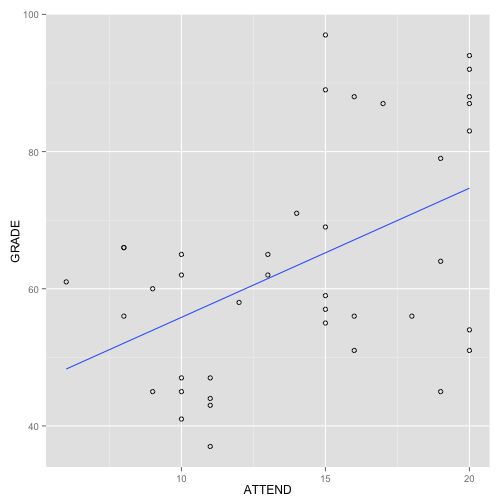Run a multiple regression in which you regress GRADE on BOOKS and ATTEND. Using the output of your analysis:

• (2a) Write the algebraic equation for this analysis as above.

• (2b) Create a graph with the same axes as 1b above. Graph the regression lines of ATTEND for students who have read 0, 2, and 4 books (so you should draw 3 lines).

``````
# Run a multiple regression in which you regress GRADE on BOOKS and ATTEND
model2 <- lm(GRADE ~ ATTEND + BOOKS, data = data)
summary(model2)
``````
``````##
## Call:
## lm(formula = GRADE ~ ATTEND + BOOKS, data = data)
##
## Residuals:
##    Min     1Q Median     3Q    Max
## -20.80 -13.37   0.06   9.17  32.29
##
## Coefficients:
##             Estimate Std. Error t value Pr(>|t|)
## (Intercept)   37.379      7.745    4.83  2.4e-05 ***
## ATTEND         1.283      0.587    2.19    0.035 *
## BOOKS          4.037      1.753    2.30    0.027 *
## ---
## Signif. codes:  0 '***' 0.001 '**' 0.01 '*' 0.05 '.' 0.1 ' ' 1
##
## Residual standard error: 14.1 on 37 degrees of freedom
## Multiple R-squared:  0.329,  Adjusted R-squared:  0.292
## F-statistic: 9.06 on 2 and 37 DF,  p-value: 0.000628
``````
``````
# Write out the algebraic equation representing this analysis
print(paste("GRADE = ", round(model2\$coeff, digits = 2), " + ", round(model2\$coeff,
digits = 2), "*ATTEND", " + ", round(model2\$coeff, digits = 2), "*BOOKS + e",
sep = ""))
``````
``````##  "GRADE = 37.38 + 1.28*ATTEND + 4.04*BOOKS + e"
``````
``````
# Create a graph with the same axes as 1b above. Graph the regression lines
# of ATTEND for students who have read 0, 2, and 4 books (so you should draw
# 3 lines).
ggplot(data, aes(x = ATTEND, y = GRADE)) + geom_point(shape = 1) + geom_abline(aes(color = "0 books"),
intercept = model2\$coeff, slope = model2\$coeff, show_guide = TRUE) +
geom_abline(aes(color = "2 books"), intercept = model2\$coeff + 2 * model2\$coeff,
slope = model2\$coeff, show_guide = TRUE) + geom_abline(aes(color = "4 books"),
intercept = model2\$coeff + 4 * model2\$coeff, slope = model2\$coeff,
show_guide = TRUE) + guides(color = guide_legend(title = "Number of books read"))
``````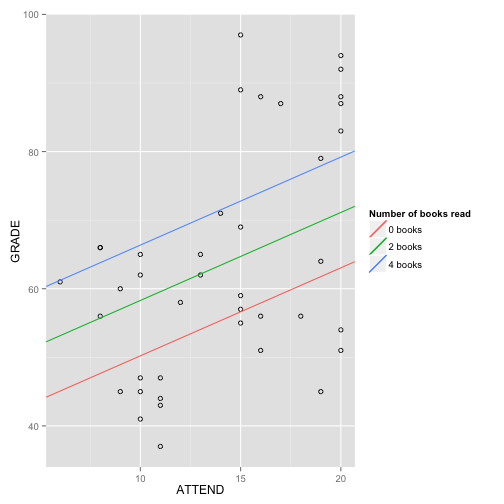Run a multiple regression in which you regress GRADE on BOOKS, ATTEND, and the interaction of BOOKS with ATTEND. Do not center any of the variables. Using the output of your analysis:

• (3a) Write the algebraic equation representing the results of this analysis three times. The first time, write it in standard form. The second time, rearrange it so that you can easily see the conditional slope of BOOKS. The third time, rearrange again so you can easily see the conditional slope of ATTEND.

• (3b) Draw a graph with GRADE on the Y-axis and ATTEND on the X-axis. Draw 3 lines depicting the regressions of ATTEND for students who have read 0, 2, and 4 books.

• (3c) Draw a graph with GRADE on the Y-axis and BOOKS on the X-axis. Draw 3 lines depicting the regressions of BOOKS for students who have attended 10, 15, and 20 times.

``````
# Run a multiple regression in which you regress GRADE on BOOKS, ATTEND, and
# the interaction of BOOKS with ATTEND
model3 <- lm(GRADE ~ ATTEND * BOOKS, data = data)
summary(model3)
``````
``````##
## Call:
## lm(formula = GRADE ~ ATTEND * BOOKS, data = data)
##
## Residuals:
##    Min     1Q Median     3Q    Max
## -24.84 -11.85   1.47   8.16  34.20
##
## Coefficients:
##              Estimate Std. Error t value Pr(>|t|)
## (Intercept)    55.221     11.262    4.90    2e-05 ***
## ATTEND         -0.137      0.878   -0.16    0.877
## BOOKS          -6.208      5.151   -1.21    0.236
## ATTEND:BOOKS    0.735      0.349    2.10    0.042 *
## ---
## Signif. codes:  0 '***' 0.001 '**' 0.01 '*' 0.05 '.' 0.1 ' ' 1
##
## Residual standard error: 13.4 on 36 degrees of freedom
## Multiple R-squared:  0.402,  Adjusted R-squared:  0.352
## F-statistic: 8.07 on 3 and 36 DF,  p-value: 0.000306
``````
``````b0 <- round(model3\$coeff, digits = 2)
attend <- round(model3\$coeff, digits = 2)
books <- round(model3\$coeff, digits = 2)
interaction <- round(model3\$coeff, digits = 2)

# Write the algebraic equation representing the results of this analysis
# three times. The first time, write it in standard form.
print(paste("GRADE = ", b0, " + ", attend, "*ATTEND", " + ", books, "*BOOKS + ",
interaction, "*ATTEND*BOOKS + e", sep = ""))
``````
``````##  "GRADE = 55.22 + -0.14*ATTEND + -6.21*BOOKS + 0.73*ATTEND*BOOKS + e"
``````
``````# The second time, rearrange it so that you can easily see the conditional
# slope of BOOKS.
print(paste("GRADE = ", b0, " + ", attend, "*ATTEND", " + (", books, " + ",
interaction, "*ATTEND)*BOOKS + e", sep = ""))
``````
``````##  "GRADE = 55.22 + -0.14*ATTEND + (-6.21 + 0.73*ATTEND)*BOOKS + e"
``````
``````# The third time, rearrange again so you can easily see the conditional
# slope of ATTEND.
print(paste("GRADE = ", b0, " + (", attend, " + ", interaction, "*BOOKS)*ATTEND + ",
books, "*BOOKS + e", sep = ""))
``````
``````##  "GRADE = 55.22 + (-0.14 + 0.73*BOOKS)*ATTEND + -6.21*BOOKS + e"
``````
``````

# Draw a graph with GRADE on the Y-axis and ATTEND on the X-axis. Draw 3
# lines depicting the regressions of ATTEND for students who have read 0, 2,
# and 4 books.
ggplot(data, aes(x = ATTEND, y = GRADE)) + geom_point(shape = 1) + geom_abline(aes(color = "0 books"),
intercept = b0, slope = attend, show_guide = TRUE) + geom_abline(aes(color = "2 books"),
intercept = b0 + 2 * books, slope = attend + 2 * interaction, show_guide = TRUE) +
geom_abline(aes(color = "4 books"), intercept = b0 + 4 * books, slope = attend +
4 * interaction, show_guide = TRUE) + guides(color = guide_legend(title = "Number of books read"))
``````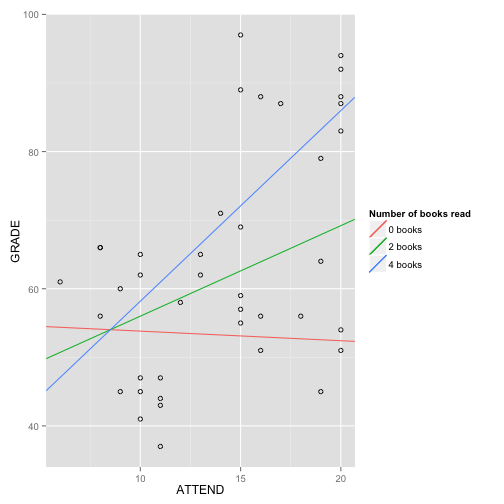``````
# Draw a graph with GRADE on the Y-axis and BOOKS on the X-axis. Draw 3
# lines depicting the regressions of BOOKS for students who have attended
# 10, 15, and 20 times.
ggplot(data, aes(x = BOOKS, y = GRADE)) + geom_point(shape = 1) + geom_abline(aes(color = "Attended 10 times"),
intercept = b0 + 10 * attend, slope = books + 10 * interaction, show_guide = TRUE) +
geom_abline(aes(color = "Attended 15 times"), intercept = b0 + 15 * attend,
slope = books + 15 * interaction, show_guide = TRUE) + geom_abline(aes(color = "Attended 20 times"),
intercept = b0 + 20 * attend, slope = books + 20 * interaction, show_guide = TRUE) +
guides(color = guide_legend(title = "Number of classes attended"))
``````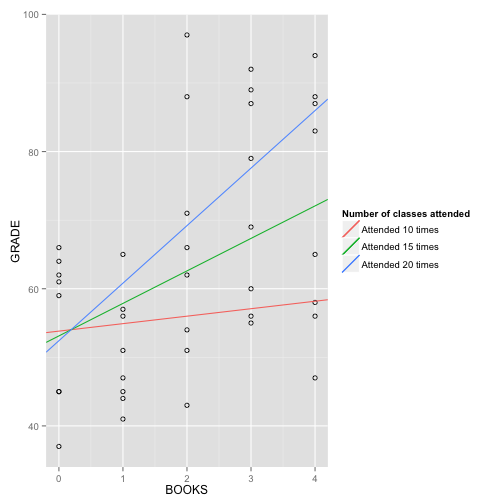Repeat the analysis you ran for #3, only this time you should first center BOOKS and ATTEND around their means, and then regress GRADE on BOOKS(centered), ATTEND(centered), and their interaction.

• (4a) Write the algebraic equation representing the results of this analysis three times. The first time, write it in standard form. The second time, rearrange it so that you can easily see the conditional slope of BOOKS. The third time, rearrange again so you can easily see the conditional slope of ATTEND.

• (4b) Draw a graph with GRADE on the Y-axis and ATTEND on the X-axis. Draw 3 lines depicting the regressions of ATTEND for students who have read 0, 2, and 4 books.

• (4c) Draw a graph with GRADE on the Y-axis and BOOKS on the X-axis. Draw 3 lines depicting the regressions of BOOKS for students who have attended 10, 15, and 20 times.

``````data\$ATTENDc <- data\$ATTEND - mean(data\$ATTEND)
data\$BOOKSc <- data\$BOOKS - mean(data\$BOOKS)

# Run a multiple regression in which you regress GRADE on BOOKS, ATTEND, and
# the interaction of BOOKS with ATTEND
model4 <- lm(GRADE ~ ATTENDc * BOOKSc, data = data)
summary(model4)
``````
``````##
## Call:
## lm(formula = GRADE ~ ATTENDc * BOOKSc, data = data)
##
## Residuals:
##    Min     1Q Median     3Q    Max
## -24.84 -11.85   1.47   8.16  34.20
##
## Coefficients:
##                Estimate Std. Error t value Pr(>|t|)
## (Intercept)      61.602      2.319   26.57   <2e-16 ***
## ATTENDc           1.333      0.562    2.37    0.023 *
## BOOKSc            4.155      1.678    2.48    0.018 *
## ATTENDc:BOOKSc    0.735      0.349    2.10    0.042 *
## ---
## Signif. codes:  0 '***' 0.001 '**' 0.01 '*' 0.05 '.' 0.1 ' ' 1
##
## Residual standard error: 13.4 on 36 degrees of freedom
## Multiple R-squared:  0.402,  Adjusted R-squared:  0.352
## F-statistic: 8.07 on 3 and 36 DF,  p-value: 0.000306
``````
``````b0 <- round(model4\$coeff, digits = 2)
attend <- round(model4\$coeff, digits = 2)
books <- round(model4\$coeff, digits = 2)
interaction <- round(model4\$coeff, digits = 2)

# Write the algebraic equation representing the results of this analysis
# three times. The first time, write it in standard form.
print(paste("GRADE = ", b0, " + ", attend, "*ATTENDc", " + ", books, "*BOOKSc + ",
interaction, "*ATTENDc*BOOKSc + e", sep = ""))
``````
``````##  "GRADE = 61.6 + 1.33*ATTENDc + 4.15*BOOKSc + 0.73*ATTENDc*BOOKSc + e"
``````
``````# The second time, rearrange it so that you can easily see the conditional
# slope of BOOKSc.
print(paste("GRADE = ", b0, " + ", attend, "*ATTENDc", " + (", books, " + ",
interaction, "*ATTENDc)*BOOKSc + e", sep = ""))
``````
``````##  "GRADE = 61.6 + 1.33*ATTENDc + (4.15 + 0.73*ATTENDc)*BOOKSc + e"
``````
``````# The third time, rearrange again so you can easily see the conditional
# slope of ATTENDc.
print(paste("GRADE = ", b0, " + (", attend, " + ", interaction, "*BOOKSc)*ATTENDc + ",
books, "*BOOKSc + e", sep = ""))
``````
``````##  "GRADE = 61.6 + (1.33 + 0.73*BOOKSc)*ATTENDc + 4.15*BOOKSc + e"
``````
``````

# Draw a graph with GRADE on the Y-axis and ATTENDc on the X-axis. Draw 3
# lines depicting the regressions of ATTENDc for students who have read 0,
# 2, and 4 booksc.
books0c <- 0 - mean(data\$BOOKS)
books2c <- 2 - mean(data\$BOOKS)
books4c <- 4 - mean(data\$BOOKS)

ggplot(data, aes(x = ATTENDc, y = GRADE)) + geom_point(shape = 1) + geom_abline(aes(color = "0 books"),
intercept = (b0 + books0c * books), slope = (attend + books0c * interaction),
show_guide = TRUE) + geom_abline(aes(color = "2 books"), intercept = (b0 +
books2c * books), slope = (attend + books2c * interaction), show_guide = TRUE) +
geom_abline(aes(color = "4 books"), intercept = (b0 + books4c * books),
slope = (attend + books4c * interaction), show_guide = TRUE) + guides(color = guide_legend(title = "Number of books read"))
``````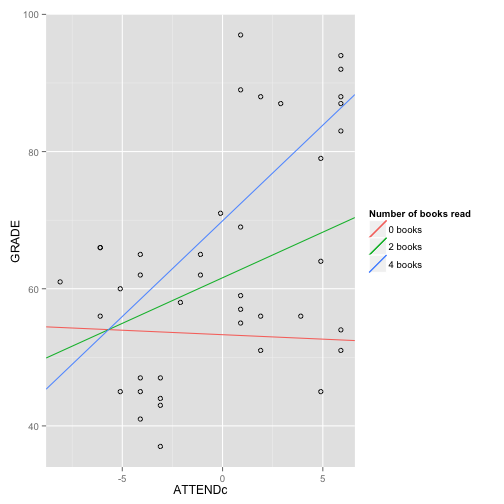``````
# Draw a graph with GRADE on the Y-axis and BOOKS on the X-axis. Draw 3
# lines depicting the regressions of BOOKS for students who have attended
# 10, 15, and 20 times.
attend10c <- 10 - mean(data\$ATTEND)
attend15c <- 15 - mean(data\$ATTEND)
attend20c <- 20 - mean(data\$ATTEND)

ggplot(data, aes(x = BOOKSc, y = GRADE)) + geom_point(shape = 1) + geom_abline(aes(color = "Attended 10 times"),
intercept = (b0 + attend10c * attend), slope = (books + attend10c * interaction),
show_guide = TRUE) + geom_abline(aes(color = "Attended 15 times"), intercept = (b0 +
attend15c * attend), slope = (books + attend15c * interaction), show_guide = TRUE) +
geom_abline(aes(color = "Attended 20 times"), intercept = (b0 + attend20c *
attend), slope = (books + attend20c * interaction), show_guide = TRUE) +
guides(color = guide_legend(title = "Number of classes attended"))
``````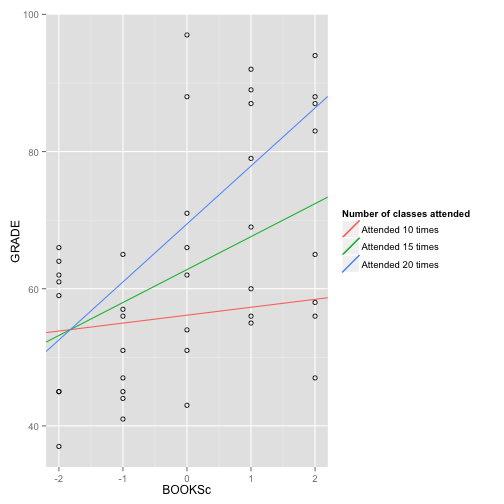Repeat #3, only this time you should first z-score all three variables (GRADE, BOOKS, and ATTEND), and then run the regression on the z-scored variables, including the product of z-BOOKS times z-ATTEND.

• (5a) Write the algebraic equation representing the results of this analysis three times. The first time, write it in standard form. The second time, rearrange it so that you can easily see the conditional slope of BOOKS. The third time, rearrange again so you can easily see the conditional slope of ATTEND.

• (5b) Draw a graph with GRADE on the Y-axis and ATTEND on the X-axis. Draw 3 lines depicting the regressions of ATTEND: one line for students who have read an average number of books, one line for students whose value on BOOKS is 1 standard deviation below the mean, and one line for students whose value on BOOKS is 1 standard deviation above the mean.

• (5c) Draw a graph with GRADE on the Y-axis and BOOKS on the X-axis. Draw 3 lines depicting the regressions of BOOKS: one line for students who have attended an average number of times, one line for students whose attendance is 1 standard deviation below the mean, and one line for students whose attendance is 1 standard deviation above the mean.

``````data\$ATTENDz <- (data\$ATTEND - mean(data\$ATTEND))/sd(data\$ATTEND)
data\$BOOKSz <- (data\$BOOKS - mean(data\$BOOKS))/sd(data\$BOOKS)

# Run a multiple regression in which you regress GRADE on BOOKSz, ATTENDz,
# and the interaction of BOOKSz with ATTENDz
model5 <- lm(GRADE ~ ATTENDz * BOOKSz, data = data)
summary(model5)
``````
``````##
## Call:
## lm(formula = GRADE ~ ATTENDz * BOOKSz, data = data)
##
## Residuals:
##    Min     1Q Median     3Q    Max
## -24.84 -11.85   1.47   8.16  34.20
##
## Coefficients:
##                Estimate Std. Error t value Pr(>|t|)
## (Intercept)       61.60       2.32   26.57   <2e-16 ***
## ATTENDz            5.70       2.40    2.37    0.023 *
## BOOKSz             5.95       2.40    2.48    0.018 *
## ATTENDz:BOOKSz     4.50       2.14    2.10    0.042 *
## ---
## Signif. codes:  0 '***' 0.001 '**' 0.01 '*' 0.05 '.' 0.1 ' ' 1
##
## Residual standard error: 13.4 on 36 degrees of freedom
## Multiple R-squared:  0.402,  Adjusted R-squared:  0.352
## F-statistic: 8.07 on 3 and 36 DF,  p-value: 0.000306
``````
``````b0 <- round(model5\$coeff, digits = 2)
attend <- round(model5\$coeff, digits = 2)
books <- round(model5\$coeff, digits = 2)
interaction <- round(model5\$coeff, digits = 2)

# Write the algebraic equation representing the results of this analysis
# three times. The first time, write it in standard form.
print(paste("GRADE = ", b0, " + ", attend, "*ATTENDz", " + ", books, "*BOOKSz + ",
interaction, "*ATTENDz*BOOKSz + e", sep = ""))
``````
``````##  "GRADE = 61.6 + 5.7*ATTENDz + 5.95*BOOKSz + 4.5*ATTENDz*BOOKSz + e"
``````
``````# The second time, rearrange it so that you can easily see the conditional
# slope of BOOKSz.
print(paste("GRADE = ", b0, " + ", attend, "*ATTENDz", " + (", books, " + ",
interaction, "*ATTENDz)*BOOKSz + e", sep = ""))
``````
``````##  "GRADE = 61.6 + 5.7*ATTENDz + (5.95 + 4.5*ATTENDz)*BOOKSz + e"
``````
``````# The third time, rearrange again so you can easily see the conditional
# slope of ATTENDz.
print(paste("GRADE = ", b0, " + (", attend, " + ", interaction, "*BOOKSz)*ATTENDz + ",
books, "*BOOKSz + e", sep = ""))
``````
``````##  "GRADE = 61.6 + (5.7 + 4.5*BOOKSz)*ATTENDz + 5.95*BOOKSz + e"
``````
``````

# Draw a graph with GRADE on the Y-axis and ATTEND on the X-axis. Draw 3
# lines depicting the regressions of ATTEND: one line for students who have
# read an average number of books, one line for students whose value on
# BOOKS is 1 standard deviation below the mean, and one line for students
# whose value on BOOKS is 1 standard deviation above the mean.
ggplot(data, aes(x = ATTENDz, y = GRADE)) + geom_point(shape = 1) + geom_abline(aes(color = "low books (-1SD)"),
intercept = b0 + -1 * books, slope = attend + -1 * interaction, show_guide = TRUE) +
geom_abline(aes(color = "ave. books (mean)"), intercept = b0, slope = attend,
show_guide = TRUE) + geom_abline(aes(color = "high books (+1SD)"), intercept = b0 +
1 * books, slope = attend + 1 * interaction, show_guide = TRUE) + guides(color = guide_legend(title = "Number of books read"))
``````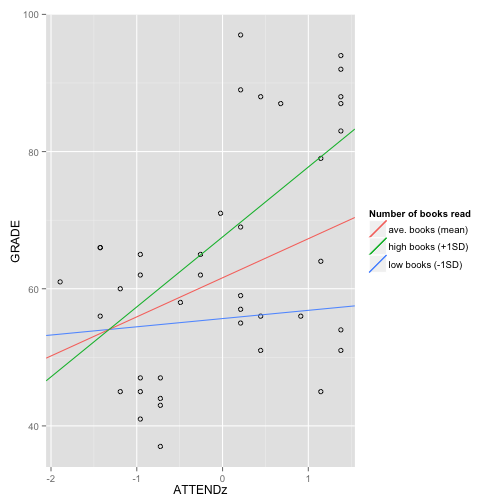``````

# Draw a graph with GRADE on the Y-axis and BOOKS on the X-axis. Draw 3
# lines depicting the regressions of BOOKS: one line for students who have
# attended an average number of times, one line for students whose
# attendance is 1 standard deviation below the mean, and one line for
# students whose attendance is 1 standard deviation above the mean.
ggplot(data, aes(x = BOOKSz, y = GRADE)) + geom_point(shape = 1) + geom_abline(aes(colour = "low attendance (-1SD)"),
intercept = b0 + -1 * attend, slope = books + -1 * interaction, size = 1,
fullrange = T, show_guide = TRUE) + geom_abline(aes(colour = "ave. attendance (mean)"),
intercept = b0, slope = books, size = 1, fullrange = T, show_guide = TRUE) +
geom_abline(aes(colour = "high attendance (+1SD)"), intercept = b0 + 1 *
attend, slope = books + 1 * interaction, size = 1, fullrange = T, show_guide = TRUE) +
guides(colour = guide_legend(title = "Attendance"))
``````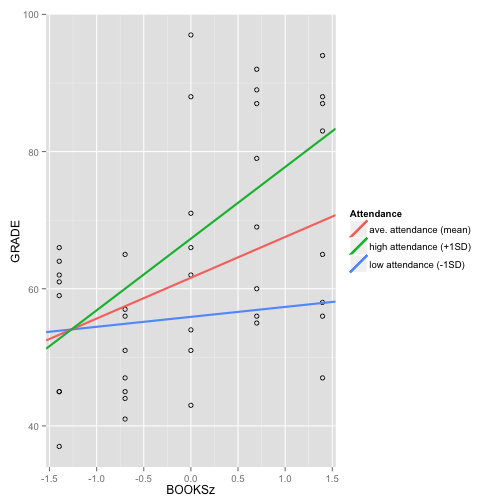Dataset source: http://www.jeremymiles.co.uk/regressionbook/data/

And John has another great way to do simple slopes in ggplot2!

I wanted to share this way of doing the simple slopes using the 'predict' function. This also demonstrates how to produce data on the fly -- good for reproducible examples!

```#Replace this with your data. # For now, making up new stuff. summary(books<-round(runif(100,from=0,to=4),0)) #Get number of books from a uniform distribution from 0-4 summary(attend<-rnorm(100,mean=14,sd=4.3)) #Get the number of days attended from a normal distribution summary(grade<-55-.137*attend-6.2*books+.74*attend*books+ #The grade is related to books and attend... rnorm(100,0,20)) #...plus unmeasured things!```

attend=attend,

#Update below is cool, but not necessary. It's just
# an easy way to make nested models. Maybe you've
# come across something like this or better?
summary(mod3<-update(mod1,.~.+books+attend:books))

#Uses a model to get predicted values for each row –
# you can use the original data or new data

require(plyr) #for the '.' function
require(ggplot2)
#I subset the data for geom_line or else we get a line for every value of books
geom_line(subset= .(books %in% c(0,2,4)), aes(colour = as.factor(books)), size = 1)+
# data. So honest!

# Surface plots!

Daryn will be going over how to make surface plots. Its just kind of a fun way to show off pretty things you can do in R. Here (rsm-plots ) is a document that covers surface plots, and here is her code!

``` #use swiss data```

``` #plot of single variable ?plot par(mfrow=c(1,2)) plot(swiss\$Education, swiss\$Fertility, main= "Graph 1", xlab= "Years of Education", ylab= "Fertility") plot(swiss\$Agriculture, swiss\$Fertility) ```

```#add a line of best fit NOT WORKING #ed.fert<-lm(swiss\$Education~swiss\$Fertility) #plot(swiss\$Education, swiss\$Fertility, main= "Graph 1", xlab= "Years of Education", ylab= "Fertility") #lines(swiss\$Education,ed.fert\$fitted) #model for Fertility as a polynomial function of Agriculture and Education swiss2.lm <- lm(Fertility ~ poly(Agriculture, Education, degree=2), data=swiss) require(rsm) #model for Fertility as a polynomial function of Agriculture and Education swiss2.lm <- lm(Fertility ~ poly(Agriculture, Education, degree=2), data=swiss) #show 3 types of graphs par(mfrow=c(1,3)) image(swiss2.lm, Education ~ Agriculture) contour(swiss2.lm, Education ~ Agriculture) persp(swiss2.lm, Education ~ Agriculture, zlab = "Fertility") #enhanced perspective plot persp(swiss2.lm, Education ~ Agriculture, col = "blue", bounds = list(Agriculture=c(20,70), Education=c(0,30)), zlab = "Predicted Fertility", contours = list(z="top", col="orange"), theta = -145, phi = 35, shade = 1) #playing with color persp(swiss2.lm, Education ~ Agriculture, col = rainbow(50), bounds = list(Agriculture=c(20,70), Education=c(0,30)), zlab = "Predicted Fertility", contours = list(z="top", col="orange"), theta = -145, phi = 35, shade = 1) jet.colors <- colorRampPalette(c("#00007F", "blue", "#007FFF", "cyan", "#7FFF7F", "yellow", "#FF7F00", "red", "#7F0000")) colorzjet <- jet.colors(100) persp(swiss2.lm, Education ~ Agriculture, col = colorzjet, bounds = list(Agriculture=c(20,70), Education=c(0,30)), zlab = "Predicted Fertility", contours = list(z="top", col="orange"), theta = -145, phi = 35, shade = 1) #saving plots - add to a plot then run whole script dev.copy(pdf,'myplot.pdf') dev.off() ```

# Totally new to R? Not for long.

Have you never used R? Nor programmed at all? The swirl package* will get you on your feet so fast. It teaches you how to use R directly from the R prompt. (* Of course, if you’re entirely new, you don’t know what a package is yet. Don’t worry! It’s sort of like an app — it wraps up a bunch of useful functions into a nice neat package.)

Get started with these instructions — they’ll walk you through installing R, R Studio, and swirl. If you need help, well, that’s what R club is for.

Here’s a snippet of what you’ll see as you run through the swirl tutorial:

```| To assign the result of `5 + 7` to a new variable
| called `x`, you type `x <- 5 + 7`. This can be
| read as "x gets 5 plus 7." Give it a try now.

> x <- 5 + 7

| You nailed it! Good job!
| You'll notice that R did not print the result
| of 12 this time. When you use the assignment
| operator, R assumes that you don't want to
| see the result immediately, but rather that
| you intend to use the result for something
| else later on.```

# Matrix algebra in R

Hi all,

To get us started off with some quick topic presentations at R Club this term, I’ll go over a little matrix algebra on Thursday. Those of you who have taken PSY613 may remember doing similar exercises in SPSS, in MATLAB, and/or by hand. Those of you who are in PSY613 currently will remember this from, well, the future, since we’ll be working on matrix algebra in lab on Friday.

Here’s a link to the code for an example: matrix_algebra.r

See you Thursday at 12pm in Franklin 271A!

``` # awesome resources: # http://www.ats.ucla.edu/stat/r/library/matrix_alg.htm # http://www.statmethods.net/advstats/matrix.html```

``` # matrix algebra in R. GLM for a t-test. ```

```?matrix() # define DV vector DV <- matrix(data=seq(1:10), nrow=10, ncol=1) # matrix(data, nrow, ncol, byrow). in this case, the data are defined by seq(1:10) # check your work. DV should be one column of 10 observations. DV class(DV) # specify design matrix. this is a t-test, so there are two groups, each n=5 # since the data for this matrix is a little harder to specify quickly, we'll do it first and then plug it into the matrix function, so it's not too messy. ?rep() group1 <- c(rep(1,5), rep(0,5)) group2 <- c(rep(0,5), rep(1,5)) X <- matrix(data=c(group1,group2), nrow=10, ncol=2) # the function matrix defaults to filling in by column (byrow=FALSE), which is what works best here. to fill in by row, byrow=TRUE # check your work. X should be two columns of 10 observations, with 1's for the first 5 observations in column 1 and 0's in column 2, and vice versa for the second 5 observations. X # GLM: DV = Xb + e. We have X and DV now, so we can solve for the parameters (betas) and the error for each observation. # assume e is zero (which is is, on average), then solve for b with X^-1 * DV n <- nrow(X) # number of observations p <- ncol(X) # number of paramaters df_m <- p-1 df_e <- n-p # X isn't square, so X^-1 is not possible. Get the pseudoinverse instead. Load MASS package to get the ginv() function library(MASS) X_inv <- ginv(X) # R defaults to element-by-element multiplication instead of matrix multiplication. Check it out: betas <- X_inv*DV # oh no! that's not right. betas <- X_inv%*%DV # yay! use %*% instead of * to specify matrix algebra. # since this is a t-test, there are two betas (2x1 matrix), one for each group mean dim(betas) # to get SS model, calculate the predicted score for each observation first model <- X%*%betas gm <- colMeans(DV) # grand mean SSM <- sum((model-gm)^2) MSM <- SSM/df_m # to get SS error, calculate error/residual for each observation (observed-predicted) errors <- DV-model SSE <- sum(errors^2) MSE <- SSE/df_e F <- MSM/MSE p_val <- 1- pf(F, df_m, df_e) ```